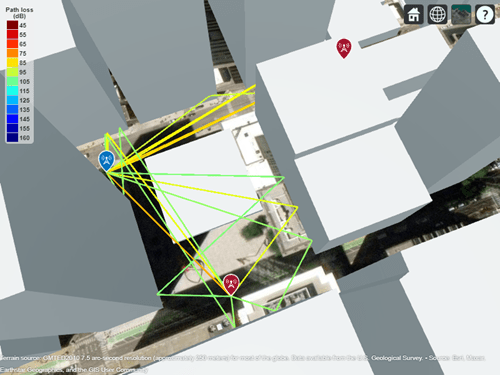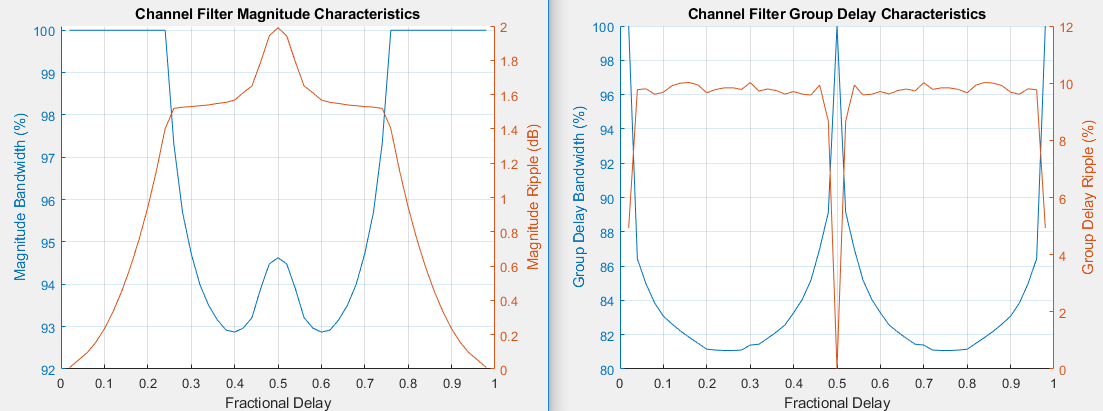# comm.ChannelFilter

Filter signal using multipath gains at specified path delays

## Description

Use the `comm.ChannelFilter` System object™ to filter a signal using multipath gains at specified path delays.

To filter a signal using multipath gains:

1. Create the `comm.ChannelFilter` object and set its properties.

2. Call the object with arguments, as if it were a function.

To learn more about how System objects work, see What Are System Objects?

## Creation

### Syntax

``chanFilt = comm.ChannelFilter``
``chanFilt = comm.ChannelFilter(Name,Value)``

### Description

````chanFilt = comm.ChannelFilter` creates a multipath channel filter System object to filter an input signal with path gains at the specified path delays```

example

````chanFilt = comm.ChannelFilter(Name,Value)` sets properties using one or more name-value pairs. For example, `'SampleRate',1e6` sets the sampling rate to `1` MHz. Enclose each property name in quotes.```

## Properties

expand all

Unless otherwise indicated, properties are nontunable, which means you cannot change their values after calling the object. Objects lock when you call them, and the `release` function unlocks them.

If a property is tunable, you can change its value at any time.

For more information on changing property values, see System Design in MATLAB Using System Objects.

Sample rate of the input signal, specified as a real, positive scalar.

Data Types: `double`

Delays of the discrete paths in seconds, specified as a real scalar or vector.

Data Types: `double`

Channel filter delay source, specified as either `'Auto'` or `'Custom'`.

• Set `FilterDelaySource` to `'Auto'` to specify the channel filter delay as the minimum possible value.

• Set `FilterDelaySource` to `'Custom'` to specify the channel filter delay as a custom value. The custom value cannot be smaller than the minimum possible value.

Data Types: `char`

Channel filter delay in samples, specified as a real, non-negative, integer scalar.

#### Dependencies

To enable this property, set the `FilterDelaySource` property to `'Custom'`. The specified value must be no smaller than the automatically determined channel filter delay when you set `FilterDelaySource` to `'Auto'`.

Data Types: `double`

Normalize outputs by number of receive antennas, specified as a logical `1` (`true`) or `0` (`false`).

Data Types: `logical`

## Usage

### Syntax

``y = chanFilt(x,g)``

### Description

example

````y = chanFilt(x,g)` filters input signal `x`, through a multipath channel with path gains `g`, at the path delay locations specified by the PathDelays property.```

### Input Arguments

expand all

Input signal, specified as a matrix. The argument `x` must be a Ns-by-Nt matrix, where Ns is the number of samples and Nt is the number of transmit antennas.

Data Types: `double`
Complex Number Support: Yes

Path gain, specified as an array. The input G must be a Ns-by-Np-by-Nt-by-Nr or 1-by-Np-by-Nt-by-Nr array, where Nr is the number of receive antennas and Np is the number of paths, i.e., the length of the PathDelays property.

Data Types: `double`
Complex Number Support: Yes

### Output Arguments

expand all

Channel output, returned as a Ns-by-Nr matrix.

Data Types: `double`
Complex Number Support: Yes

## Object Functions

To use an object function, specify the System object as the first input argument. For example, to release system resources of a System object named `obj`, use this syntax:

`release(obj)`

expand all

 `info` Return characteristic information about channel filter
 `step` Run System object algorithm `release` Release resources and allow changes to System object property values and input characteristics `reset` Reset internal states of System object

## Examples

collapse all

In a distributed MIMO system, explore spatial diversity by transmitting the same signal from two geographically separated transmitters and combining the received signals at one receiver. Use ray tracing to analyze the propagation paths and gains from each transmitter to receiver.

Perform Ray Tracing

Import buildings data for Chicago into `siteviewer` from an OpenStreetMap® (osm) file. For more information about the osm file, see . Place two transmitter and one receiver sites in the city.

```sv = siteviewer("buildings","Chicago.osm"); rx = rxsite("Name","Receiver", ... "Latitude",41.878543,"Longitude",-87.630599, ... "AntennaHeight",1.5); show(rx); tx1 = txsite("Name","Transmitter #1", ... "Latitude",41.878996,"Longitude",-87.629361); show(tx1); tx2 = txsite("Name","Transmitter #2", ... "Latitude",41.880142,"Longitude",-87.630850); show(tx2);```

Perform ray tracing from each transmitter site to the receiver site with up to second order reflection. Plot the computed rays.

```rays = raytrace([tx1, tx2],rx); plot([rays{:}]);```Ray tracing finds several ray paths to the receiver from each transmitter. From the map we can visually see the first transmitter is closer to the receiver than the second transmitter. We can also see the first transmitter has more reflected paths to the receiver. Display the propagation delays associated with each transmitter.

`pd1 = [rays{1}.PropagationDelay]`
```pd1 = 1×10 10-6 × 0.3830 0.3839 0.5476 0.6482 0.5485 0.5486 0.6572 0.6945 0.7140 0.8874 ```
`pd2 = [rays{2}.PropagationDelay]`
```pd2 = 1×5 10-6 × 0.5967 0.5973 0.6059 0.6066 0.6255 ```

Construct one channel filter for each transmitter site. Specify a sample rate of 30 MHz and use the minimum delay among the seven rays as the reference of time 0.

```chanFilt1 = comm.ChannelFilter( ... "SampleRate",30e6, ... "PathDelays",pd1-min([pd1, pd2]))```
```chanFilt1 = comm.ChannelFilter with properties: SampleRate: 30000000 PathDelays: [0 8.7598e-10 1.6459e-07 2.6516e-07 1.6553e-07 1.6565e-07 2.7417e-07 3.1151e-07 3.3105e-07 5.0442e-07] FilterDelaySource: 'Auto' NormalizeChannelOutputs: true ```
```chanFilt2 = comm.ChannelFilter( ... "SampleRate",30e6, ... "PathDelays",pd2-min([pd1, pd2]))```
```chanFilt2 = comm.ChannelFilter with properties: SampleRate: 30000000 PathDelays: [2.1372e-07 2.1434e-07 2.2294e-07 2.2357e-07 2.4247e-07] FilterDelaySource: 'Auto' NormalizeChannelOutputs: true ```

The individual channel filters for the two transmitters yield different filter delay values. Use the `info` object function of `comm.ChannelFilter` to show the filter delay of the two channel filters.

`fd1 = chanFilt1.info.ChannelFilterDelay`
```fd1 = 7 ```
`fd2 = chanFilt2.info.ChannelFilterDelay`
```fd2 = 1 ```

The two channel filters must have the same filter delay to combine the channel outputs at the receiver site. Customize the filter delay for each channel filter to use the larger value of the individually computed delay values.

```set(chanFilt1,"FilterDelaySource","Custom", ... "FilterDelay",max(fd1,fd2)); set(chanFilt2,"FilterDelaySource","Custom", ... "FilterDelay",max(fd1,fd2));```

Apply Receive Signal Combining

Set up system parameters, assigning only one isotropic antenna at each site.

```Nt = 1; % Number of transmit elements Ns = 1000; % Samples per frame M = 64; % Modulation order ```

Retrieve path gains from the computed rays. Assume the sites are static and no Doppler shift is introduced.

```pg1 = 10.^(-[rays{1}.PathLoss]/20) .* ... exp(1i*[rays{1}.PhaseShift]); pg2 = 10.^(-[rays{2}.PathLoss]/20) .* ... exp(1i*[rays{2}.PhaseShift]);```

Generate a frame of random 64-QAM signals. Perform channel filtering for each transmitter site and receive signal combining. The combined 2x1 distributed MIMO channel has a filter delay of max(`fd1`,`fd2`).

```x = qammod(randi([0, M-1],Ns,Nt),M); y = chanFilt1(x,pg1) + chanFilt2(x,pg2);```

Appendix

Construct a channel filter object with the LTE Extended Vehicular A model (EVA) delay profile.

```chanFilt = comm.ChannelFilter( ... 'SampleRate', 30.72e6, ... 'PathDelays', [0 30 150 310 370 710 1090 1730 2510]*1e-9);```

Set up system parameters. There are two transmit and receive antennas.

```[Nt, Nr] = deal(2); Ns = 30720; Np = length(chanFilt.PathDelays); M = 256;```

Generate random 256-QAM signal and complex path gains.

```x = qammod(randi([0, M-1], Ns, Nt), M); g = complex(rand(Ns, Np, Nt, Nr), rand(Ns, Np, Nt, Nr));```

Filter the signal with path gains for the EVA delay profile.

`y = chanFilt(x, g);`

Using one MIMO channel System object™ and two identically configured channel filter System objects, switch a link-level simulation between 3-by-2 downlink and reciprocal 2-by-3 uplink signal transmissions.

Define system parameters.

```modOrder = 256; % Modulation order Nant1 = 3; % Number of 'transmit' antennas Nant2 = 2; % Number of 'receive' antennas Rs = 1e6; % Sample rate pd = [0 1.5 2.3]*1e-6; % Path delays frmLen = 1e3; % Frame length```

Create a MIMO channel System object™, configuring it for path gain generation by disabling channel filtering.

```chan = comm.MIMOChannel( ... 'SampleRate',Rs, ... 'PathDelays',pd, ... 'AveragePathGains',[1.5 1.2 0.2], ... 'MaximumDopplerShift',300, ... 'SpatialCorrelationSpecification','none', ... 'NumTransmitAntennas',Nant1, ... 'NumReceiveAntennas',Nant2, ... 'ChannelFiltering',false, ... 'NumSamples',frmLen);```

Create identical channel filter System objects for both transmission directions: one channel filter for the `Nant1`-by-`Nant2` downlink channel (3 transmit antennas to 2 receive antennas) and a reciprocal channel filter for the `Nant2`-by-`Nant1` uplink channel (2 transmit antennas to 3 receive antennas).

```chanFiltDownlink = comm.ChannelFilter( ... 'SampleRate',Rs, ... 'PathDelays',pd); chanFiltUplink = clone(chanFiltDownlink);```

Generate random path gains for one frame of the downlink 3-by-2 channel. Pass randomly generated 256-QAM signals through the 3-by-2 downlink channel.

```pgDownlink = chan(); x = qammod(randi([0 modOrder-1],frmLen,Nant1),modOrder); yDL = chanFiltDownlink(x,pgDownlink);```

Switch the link direction. Run the channel object to generate another frame of path gains, permuting its 3rd (Tx) and 4th (Rx) dimensions for the reciprocal uplink 2-by-3 channel. Pass randomly generated 256-QAM signals through the 2-by-3 reciprocal uplink channel.

```pgUplink = permute(chan(),[1 2 4 3]); x = qammod(randi([0 modOrder-1],frmLen,Nant2),modOrder); yUL = chanFiltUplink(x,pgUplink);```

Show the sizes of the downlink and uplink path gain arrays returned by the MIMI channel object as an ${\mathit{N}}_{\mathrm{S}}$-by-${\mathit{N}}_{\mathrm{P}}$-by-${\mathit{N}}_{\mathrm{T}}$-by-${\mathit{N}}_{\mathrm{R}}$ array.

• ${\mathit{N}}_{\mathrm{S}}$ is the number of samples.

• ${\mathit{N}}_{\mathrm{P}}$ is the number of path delays.

• ${\mathit{N}}_{\mathrm{T}}$ is the number of transmit antennas. `Nant1` for downlink and `Nant2` for uplink.

• ${\mathit{N}}_{\mathrm{R}}$ is the number of receive antennas. `Nant2` for downlink and `Nant1` for uplink.

`size(pgDownlink)`
```ans = 1×4 1000 3 3 2 ```
`size(pgUplink)`
```ans = 1×4 1000 3 2 3 ```

Show the size of the channel output matrices returned by the MIMI channel object as an ${\mathit{N}}_{\mathrm{S}}$-by-${\mathit{N}}_{\mathrm{R}}$ matrix. ${\mathit{N}}_{\mathrm{S}}$ is the number of samples. ${\mathit{N}}_{\mathrm{R}}$ is the number of receive antennas.

`size(yDL)`
```ans = 1×2 1000 2 ```
`size(yUL)`
```ans = 1×2 1000 3 ```

## Algorithms

The channel filter implements a fractional delay (FD) finite impulse response (FIR) bandpass filter with a length of 16 coefficients for each candidate fractional delay at 0, 0.02, 0.04, …, 0.98.

Each discrete path is rounded to its nearest candidate fractional delay, so the delay error limit is 1% of the sample time. To achieve a group delay bandwidth exceeding 80% and a magnitude bandwidth exceeding 90%, the algorithm selects the optimal FIR coefficient values for each fractional delay, while satisfying the following criteria:

• Group delay ripple ≤ 10%

• Magnitude ripple ≤ 2 dB

• Magnitude bandedge attenuation = 3 dB

The plots show bandwidths that satisfy the design criteria for group delay ripple, magnitude ripple, and magnitude bandedge attenuation.For additional information, see the article A Matlab-based Object-Oriented Approach to Multipath Fading Channel Simulation at MATLAB® Central.

## Version History

Introduced in R2020b